# Ratio of sides 2

The ratio of the side lengths of one square to another is 1:2. Find the ratio of the area of the two squares.

r =  1:4

### Step-by-step explanation: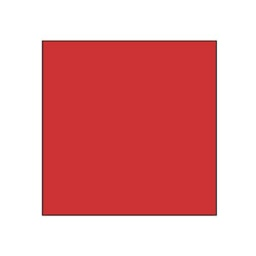Did you find an error or inaccuracy? Feel free to write us. Thank you!Tips to related online calculators
Check out our ratio calculator.

#### You need to know the following knowledge to solve this word math problem:

We encourage you to watch this tutorial video on this math problem:

## Related math problems and questions:

• Square area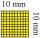Complete the table and then draw each square. Provide exact lengths. Describe any problems you have. Side Length Area Square 1 1 unit2 Square 2 2 units2 Square 3 4 units2
• Similarity of squares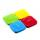The ratio of the similarity of the squares ABCD and KLMN is 2.5. Square KLMN area is greater than area of a square ABCD with side a: ?
• Two squaresTwo squares whose sides are in the ratio 5:2 have a sum of its perimeters 73 cm. Calculate the sum of the area of these two squares.
• In theIn the national park, the ratio of the wooded area to grassland is 4: 1. The total area is 385km2. What area is wooded?
• Squares above sidesTwo squares are constructed on two sides of the ABC triangle. The square area above the BC side is 25 cm2. The height vc to the side AB is 3 cm long. The heel P of height vc divides the AB side in a 2: 1 ratio. The AC side is longer than the BC side. Calc
• Line segmentCut a line segment of 15 cm into two line segments so that their lengths are in ratio 2:1. What length will each have?
• ExpansionIf one side of the rectangle is larger 4-times and second 2-times, what percentage increases the area of rectangle?
• A perimeter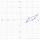A perimeter of a parallelogram is 2.8 meters. The length of one of its sides is equal to one-seventh of the entire perimeter. Find lengths of the sides of the parallelogram.
• Land areaThe land area of Asia and Africa are in a 3: 2 ratio, the European and African are is 1:3. What are the proportions of Asia, Africa, and Europe?
• Trapezoid RTThe plot has a shape of a rectangular trapezium ABCD, where ABIICD with a right angle at the vertex B. side AB has a length of 36 m. The lengths of the sides AB and BC are in the ratio 12:7. Lengths of the sides AB and CD are a ratio of 3:2. Calculate con
• Square to rectangle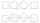What is the ratio of the area of a square of side x to the area of a rectangle of a rectangle of width 2 x and length 3
• Composite ratioJakub, Aneta, and Lenka divided 1342 USD in the ratio 5/2: 3/10: 1/4. How much did Lenka take?
• DonutsFind how many donuts each student will receive if you share 126 donuts in a ratio of 1:5:8
• GardenThe area of a square garden is 2/9 of triangle garden with sides 160 m, 100 m, and 100 m. How many meters of fencing need to fence a square garden?
• Two brothersThe two brothers were to be divided according to the will of land at an area of 1ha 86a 30m2 in a ratio of 5:4. How many will everyone get?
• InfinityIn a square with side 19 is inscribed circle, the circle is inscribed next square, again circle, and so on to infinity. Calculate the sum of the area of all these squares.
• GlassAt the glass shop we have to cut 8 sheets of glass. Each was shaped a square with sides of 18 cm. We paid 44 CZK. How much is a 1 m2 of glass?